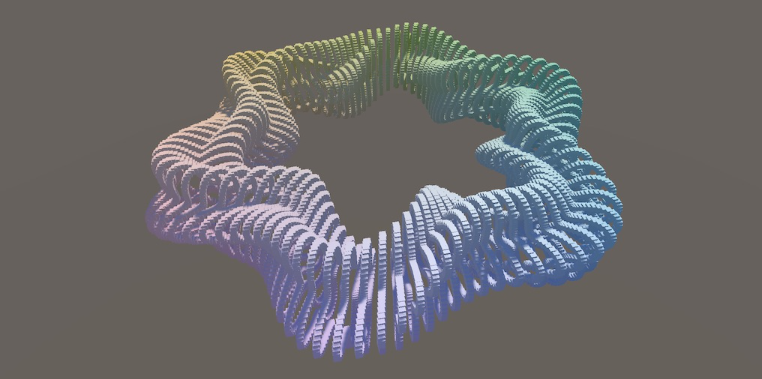# 前言

•  创建数学函数库
•  使用委托和枚举类型
•  使用网格显示 2D 函数
•  在 3D 空间中定义曲面# 函数库

## 创建库基类(Library Class)

using UnityEngine;
public class FunctionLibrary {}


using UnityEngine;
public static class FunctionLibrary {}


## 函数方法

public static class FunctionLibrary {
void Wave()
{

}
}


public static class FunctionLibrary {
static void Wave()
{

}
}


public static class FunctionLibrary {
public static void Wave()
{

}
}


public static float Wave()
{

}


public static float Wave(float x,float t)
{
Mathf.Sin(Mathf.PI*(x + t));
}


public static float Wave(float x,float t)
{
return Mathf.Sin(Mathf.PI*(x + t));
}


position.y = FunctionLibrary.Wave(position.x,time);  //计算新的位置


## 隐式的使用类型

using UnityEngine;
using static UnityEngine.Mathf;

public static class FunctionLibrary {
public static float Wave(float x,float t)
{
return Mathf.Sin(Mathf.PI*(x + t));
}
}


return Sin(PI*(x + t));


## 添加新的函数方法

Q.E.D.

••